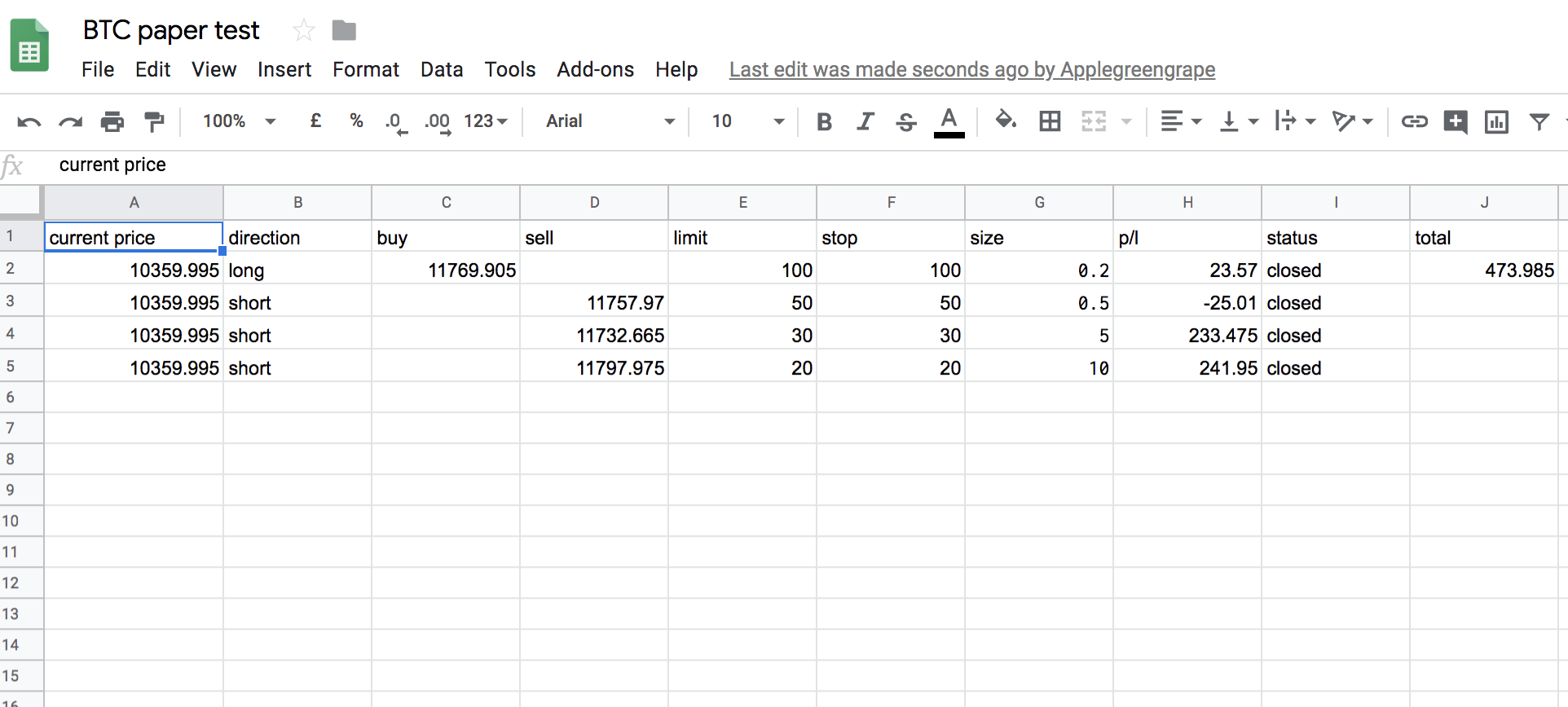I was inspired by the alpaca’s idea of using google spreadsheet as a test environment for auto algo trading. So I decided to make a version for bitcoin paper test trading. For alpaca’s stock version, you can find the details here. The google spreadsheet will look like this:I am using coinbase api to get the lastest Bitcoin price. And there is already one google app script class that you can use - `Class UrlFetchApp`. Then you need to call this class in your function.

``````function myFunction() {
// Make a GET request and log the returned content.
var response = UrlFetchApp.fetch('https://api.coinbase.com/v2/prices/BTC-USD/spot');
var fact = response.getContentText();
var json = JSON.parse(fact)

Logger.log(json.data.amount)
var lastRow = sheet.getLastRow();

for (var i = 2; i <= lastRow; i++){
// update each row to set the latest bitcoin price
sheet.getRange(i,1).setValue([json.data.amount]);

}
...
}
``````

Now we need to do some small calculation to update the position status (open, close and closed profit/loss). We can use the class sheet. Then the script will be some thing like this:

``````function myFunction() {
...
for (var i = 2; i <= lastRow; i++ ){

var status = sheet.getRange(i,9).getValues();
var price = sheet.getRange(i,1).getValues();
var direction = sheet.getRange(i,2).getValues();
var sell = sheet.getRange(i,4).getValues();
var limit = sheet.getRange(i,5).getValues();
var stop = sheet.getRange(i,6).getValues();
var size = sheet.getRange(i,7).getValues();

if ( status == "closed"){}
else {

//  Logger.log("still open")
sheet.getRange(i,9).setValue("open");
sheet.getRange(i,8).setValue(pl);

}else{
// Logger.log("closed")
sheet.getRange(i,9).setValue("closed");
sheet.getRange(i,8).setValue(pl);

}}

else{
if( direction =="short" ){
if(sell-price<limit && price-sell<stop){
//  Logger.log("still open")
sheet.getRange(i,9).setValue("open");
var pl = (sell-price)*size
sheet.getRange(i,8).setValue(pl);

}else{
// Logger.log("closed")
sheet.getRange(i,9).setValue("closed");
var pl = (sell-price)*size
sheet.getRange(i,8).setValue(pl);
}
}
}
}
}
}
``````

You can find the source code here. Please feel free to 👉📱message my twilio bot +447479275693. I will come back to you shortly 😃.